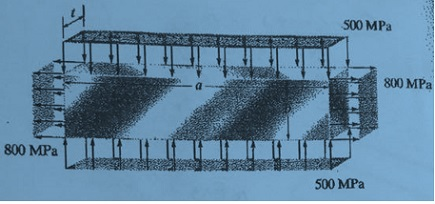# The copper bar is subjected to uniform loading along its edges as shown. If the bar has length a...

## Question:The copper bar is subjected to uniform loading along its edges as shown. If the bar has length a = 300 mm, width b = 50 mm, and thickness t = 20 mm before the load is applied, determine its new length,width and thickness after application of the load. Take {eq}E_{cu}=120 GPa {/eq}

## Hooke's Law:

Hooke's Law relates the stress with the deformations or strains up to a certain elastic limit. The general expression for Hooke's law is {eq}\sigma \propto \varepsilon {/eq}, where the stress is {eq}\sigma {/eq} and the strain is {eq}\varepsilon {/eq}. Hooke's law is applicable for the ductile material under the elastic limit. This law also derives the spring force.

Given Data

• The normal tensile stress along horizontal direction is: {eq}{\sigma _x} = 800\;{\rm{MPa}} {/eq}
• The normal compressive stress along...

Become a Study.com member to unlock this answer! Create your account